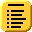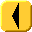## Introduction to Programming and C++Suppose we have a base class Base containing a protected member variable base. By the rules of inheritance, this variable would be inherited and accessible to any derived class, as displayed in Program 1.

Program 1. Accessing a base protected member variable from a derived class.

```
#include <iostream>
using namespace std;

class Base {
protected:
int base;
public:
Base( int m ):base( m ) { }
};

class Derived:public Base {
public:
Derived( int n ):Base( n ) { }

void display() const {
cout << "The base contains: " << base << endl;
}
};

int main() {
Derived s(3);
s.display();
return 0;
}
```

If the base class is templated, but the derived class inherits from base class where the template variable is specified, the derived class continues to have access to the base class protected member variables, as shown in Program 2.

Program 2. Accessing a base protected member variable from a derived class where the base class is templated but the derived class is not.

```
#include <iostream>
using namespace std;

template <typename T>
class Base {
protected:
T base;
public:
Base( const T &t ):base( t ) { }
};

class Derived:public Base<int> {
public:
Derived( int n ):Base<int>( n ) { }

void display() const {
cout << "The base contains: " << base << endl;
}
};

int main() {
Derived s(3);
s.display();
return 0;
}
```

Unfortunately, if the derived class also supports the template, you have code which does not compile:

Program 3. The derived class cannot access the base class protected member variable.

```
#include <iostream>
using namespace std;

template <typename T>
class Base {
protected:
T base;
public:
Base( const T &t ):base( t ) { }
};

template <typename T>
class Derived:public Base<T> {
public:
Derived( T n ):Base<T>( n ) { }

void display() const {
// THIS DOES NOT WORK
cout << "The base contains: " << base << endl;
}
};

int main() {
Derived<int> s(3);
s.display();
return 0;
}
```

Programs 4, 5, and 6 show three ways of accessing the protected member variables of the base class, the first specifying the scope, the second using using, and the third explicitly using this.

Program 4. Accessing the base protected member variables by providing the scope.

```
template <typename T>
class Derived:public Base<T> {
public:
Derived( T n ):Base<T>( n ) { }

void display() const {
cout << "The base contains: " << Base<T>::base << endl;
}
};
```

Program 5. Accessing the base protected member variables by using the using keyword.

```
template <typename T>
class Derived:public Base<T> {
using Base<T>::base;

public:
Derived( T n ):Base<T>( n ) { }

void display() const {
cout << "The base contains: " << base << endl;
}
};
```

Program 6. Accessing the base protected member variables by using this.

```
template <typename T>
class Derived:public Base<T> {
public:
Derived( T n ):Base<T>( n ) { }

void display() const {
cout << "The base contains: " << this->base << endl;
}
};
```

If you think that this is a bug in C++, it is not: please read the following comments http://gcc.gnu.org/gcc-3.4/changes.html with the bullet starting with In a template definition, unqualified names will no longer find members of a dependent base....Top